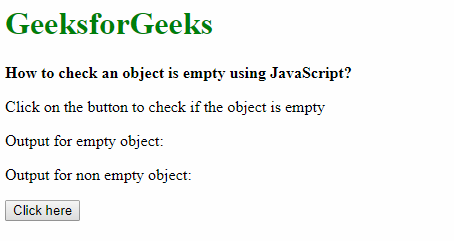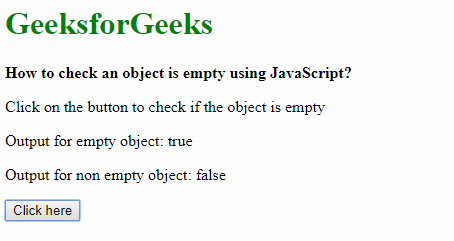# How to check an object is empty using JavaScript?

Method 1: Using the Object.keys(object) method: The required object could be passed to the Object.keys(object) method which will return the keys in the object. The length property is used to the result to check the number of keys. If the length property returns 0 keys, it means that the object is empty.

Syntax:

`Object.keys(object).length === 0`

Example:

 ` ` `<``html``> ` `     `  `<``head``> ` `    ``<``title``> ` `        ``How to check an object is  ` `        ``empty using JavaScript? ` `    `` ` ` ` ` `  `<``body``> ` `    ``<``h1` `style``=``"color: green"``> ` `        ``GeeksforGeeks ` `    `` ` `     `  `    ``<``b``> ` `        ``How to check an object is ` `        ``empty using JavaScript? ` `    `` ` `     `  `    ``<``p``> ` `        ``Click on the button to check ` `        ``if the object is empty ` `    `` ` `     `  `    ``<``p``>Output for empty object:  ` `        ``<``span` `class``=``"outputEmpty"``> ` `    `` ` `     `  `    ``<``p``>Output for non empty object:  ` `        ``<``span` `class``=``"outputNonEmpty"``> ` `    `` ` ` `  `    ``<``button` `onclick``=``"checkObject()"``> ` `        ``Click here ` `    `` ` `     `  `    ``` `    ``<``script` `type``=``"text/javascript"``> ` `        ``function checkObject() { ` `             `  `            ``let emptyObj = {} ` `            ``let nonEmptyObj = { ` `                ``title: 'Title 1', ` `                ``info: 'Sample Info' ` `            ``} ` ` `  `            ``ans1 = (Object.keys(emptyObj).length === 0) ` `            ``document.querySelector('.outputEmpty').textContent ` `                    ``= ans1; ` ` `  `            ``ans2 = (Object.keys(nonEmptyObj).length === 0) ` `            ``document.querySelector('.outputNonEmpty').textContent ` `                    ``= ans2; ` `        ``} ` `    `` ` ` ` ` `  `                     `

Output:

• Before clicking the button:• After clicking the button:Method 2: Looping through the object using object.hasOwnProperty(key): A function is created where the object is looped over and checked if it contains the ‘key’ property using the object.hasOwnProperty() method. This function would return true if it could find no keys in the loop, which means that the object is empty. If any key is encountered, the loop breaks and false is returned. This method also works for older browsers that may not support the first method.

Syntax:

```function isEmptyObj(object) {
for (var key in object) {
if (object.hasOwnProperty(key)) {
return false;
}
}
}
```

Example:

 ` ` `<``html``> ` `     `  `<``head``> ` `    ``<``title``> ` `        ``How to check an object is ` `        ``empty using JavaScript? ` `    `` ` ` ` ` `  `<``body``> ` `    ``<``h1` `style``=``"color: green"``> ` `        ``GeeksforGeeks ` `    `` ` `     `  `    ``<``b``> ` `        ``How to check an object is ` `        ``empty using JavaScript? ` `    `` ` `     `  `    ``<``p``> ` `         ``on the button to check ` `         ``if the object is empty ` `    `` ` `     `  `    ``<``p``> ` `        ``Output for empty object:  ` `        ``<``span` `class``=``"outputEmpty"``> ` `    `` ` `     `  `    ``<``p``> ` `        ``Output for non empty object:  ` `        ``<``span` `class``=``"outputNonEmpty"``> ` `    `` ` `  `  `    ``<``button` `onclick``=``"checkObject()"``> ` `        ``Click here ` `    `` ` `     `  `    ``<``script` `type``=``"text/javascript"``> ` `  `  `        ``function checkObject() { ` `            ``let emptyObj = {} ` `            ``let nonEmptyObj = { ` `                ``title: 'Title 1', ` `                ``info: 'Sample Info' ` `            ``} ` `  `  `            ``ans1 = isEmptyObj(emptyObj); ` `            ``document.querySelector('.outputEmpty').textContent ` `                    ``= ans1; ` `  `  `            ``ans2 = isEmptyObj(nonEmptyObj); ` `            ``document.querySelector('.outputNonEmpty').textContent ` `                    ``= ans2; ` `        ``} ` `  `  `        ``function isEmptyObj(object) { ` `            ``for (var key in object) { ` `                ``if (object.hasOwnProperty(key)) { ` `                    ``return false; ` `                ``} ` `            ``} ` `  `  `            ``return true; ` `        ``} ` `    `` ` ` ` ` `  ` `

Output:

• Before clicking the button:• After clicking the button:My Personal Notes arrow_drop_upCheck out this Author's contributed articles.

If you like GeeksforGeeks and would like to contribute, you can also write an article using contribute.geeksforgeeks.org or mail your article to contribute@geeksforgeeks.org. See your article appearing on the GeeksforGeeks main page and help other Geeks.

Please Improve this article if you find anything incorrect by clicking on the "Improve Article" button below.